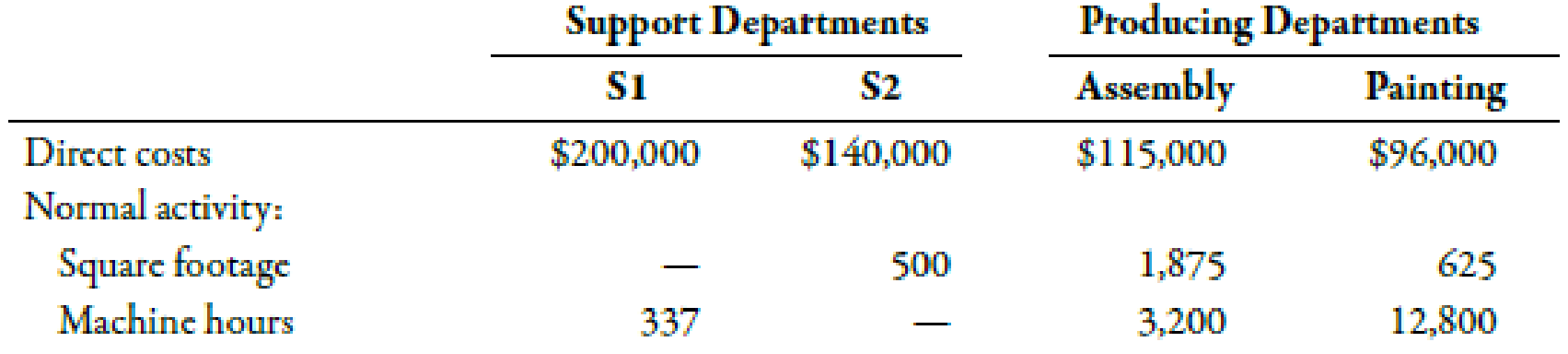Chapter 4, Problem 35BEB### Managerial Accounting: The Corners...

7th Edition
Maryanne M. Mowen + 2 others
ISBN: 9781337115773

#### Solutions

Chapter
Section### Managerial Accounting: The Corners...

7th Edition
Maryanne M. Mowen + 2 others
ISBN: 9781337115773
Textbook Problem
11 views

# Use the following information for Brief Exercises 4-34 and 4-35:Sanjay Company manufactures a product in a factory that has two producing departments, Assembly and Painting, and two support departments, S1 and S2. The activity driver for S1 is square footage, and the activity driver for S2 is number of machine hours. The following data pertain to Sanjay:Brief Exercises 4-35 (Appendix 4B) Sequential MethodRefer to the information for Sanjay Company on the previous page. Now assume that Sanjay uses the sequential method to allocate support department costs. S1 is allocated first, then S2.Required: 1. Calculate the cost assignment ratios to be used under the sequential method for S2, Assembly, and Painting. Carry out your answers to four decimal places. 2. Allocate the overhead costs to the producing departments by using the sequential method.

1.

To determine

Computecost assignment ratios for S2 under sequential method.

Explanation

Sequential Method:

Sequential method recognizes that there is possible interaction between the support departments. However, it does notaccount for such interaction in full which makes it more accurate as compared to the direct method.

Use the following formula to calculatecost assignment ratios for S1 on the basis of number of square footage:

S2:

S2=Numberofsquare footageinS2Totalsquare footage

Substitute 500 for number of square footage in S2 and 3,000 for total square footage in the above formula.

S2=500square footage3,000square footage=0.1667

Therefore, the cost assignment ratio for S2 is 0.1667.

Assembly department:

Assembly=Numberofsquare footageincuttingTotalsquare footage

Substitute 1,875 for number ofsquare footage in assembly and 3,000 for total square footage in the above formula.

Assembly=1,875square footage3,000square footage=0.6250

Therefore, the cost assignment ratio for assembly department is 0.6250.

Painting department:

Painting=Numberofsquare footageinsewingTotalsquare footage

Substitute 625 for number ofsquare footage in assembly and 3,000 for total square footage in the above formula.

Painting=625square footage3,000square footage=0.2083

Therefore, the cost assignment ratio for painting department is 0.2083.

Use the following formula to calculate cost assignment ratios for Department S2 on the basis of number of machine hours:

Assembly department:

Assembly=Numberofmachine hoursofassemblyTotalmachine hoursofproducingdepartments

Substitute 3,200 for number of machine hoursofassembly and 16,000 for total machine hours of producing department in the above formula.

Assembly=3,200hours16,000hours=0

2.

To determine

Allocate the support department costs to the producing departments by using the sequential method:

### Still sussing out bartleby?

Check out a sample textbook solution.

See a sample solution

#### The Solution to Your Study Problems

Bartleby provides explanations to thousands of textbook problems written by our experts, many with advanced degrees!

Get Started